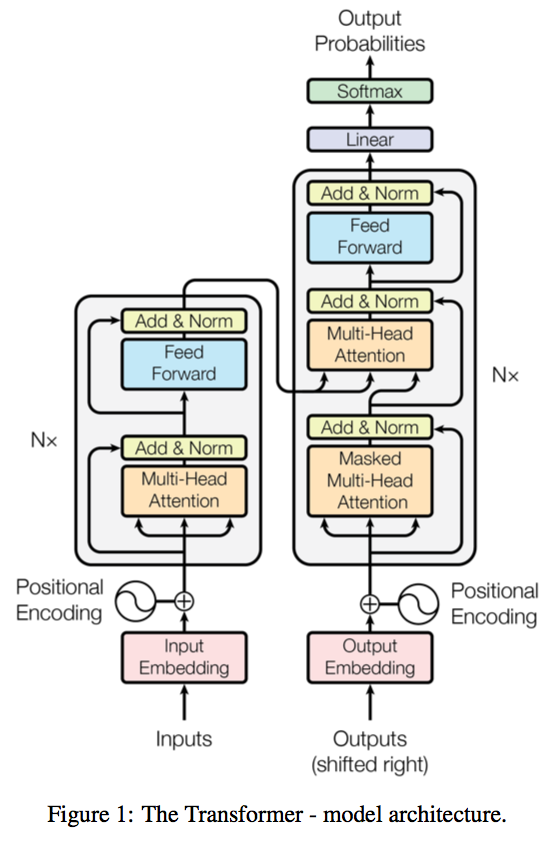# Transformer 个人小结

transformer 已经出来三年了，自己也没有深入了解，触及皮毛，最近想研究一下detr, 顺便写个 📒 记录一下对transformer的理解，个人主要从代码角度理解它的训练、测试的输入输出，关于理论网上太多了，自己只记录几个重点。这篇博客主要参考于李宏毅教授的课程以及 pytorch的transformer代码。

## self-attention## 位置编码def _get_sinusoid_encoding_table(self, n_position, d_hid):
def get_position_angle_vec(position):
return [position / np.power(10000, 2 * (hid_j // 2) / d_hid) for hid_j in range(d_hid)]
sinusoid_table = np.array([get_position_angle_vec(pos_i) for pos_i in range(n_position)])
sinusoid_table[:, 0::2] = np.sin(sinusoid_table[:, 0::2])
sinusoid_table[:, 1::2] = np.cos(sinusoid_table[:, 1::2])
return torch.FloatTensor(sinusoid_table).unsqueeze(0)



## FFN

class PositionwiseFeedForward(nn.Module):
''' A two-feed-forward-layer module '''

def __init__(self, d_in, d_hid, dropout=0.1):
super().__init__()
self.w_1 = nn.Linear(d_in, d_hid) # position-wise
self.w_2 = nn.Linear(d_hid, d_in) # position-wise
self.layer_norm = nn.LayerNorm(d_in, eps=1e-6)
self.dropout = nn.Dropout(dropout)

def forward(self, x):

residual = x

x = self.w_2(F.relu(self.w_1(x)))
x = self.dropout(x)
x += residual

x = self.layer_norm(x)

return x



## transformer结构src_mask: 输入mask, 对于每个句子 src_mask 是个一维bool向量，对于batch训练的输入，各个句子的长短不一，需要对短句子进行填充，但是计算attention的时候是整个句子计算，所有不希望对填充的token计算attention值，src_mask 标志哪些是填充token，计算attention时在softmax前后赋予其很小的值。

trg_mask: 目标句子的mask, 对于每个句子，句子有n个单词，trg_mask 是个 n * n 的矩阵，比如在目标语句中第一个单词只能和自己计算attention值，第二个单词能看见第一个单词，所以可以与第一个单词和自己计算attention值，以此类推，seq2seq 模型在解码器训练和测试时一般会输入一个单词预测下一个，然后将预测的单词的再输入解码器预测下一个，测试时只能这样，但训练时这样就存在串行，效率低。因此transformer在训练时采用teacher forcing策略，直接将整个gt句子用于decoder的输入，那么一次性进行整个句子的预测，计算每个预测单词的loss.

|true | false | false|
|true | true  | false|
|true | true  | true |


Masked Multi-Head Self-attention : 在decoder中，第一次是Masked Multi-Head Self-attention 层，因为训练时输入了整个句子的ground-truth，那么会计算各个单词之间的attention值，但是实际预测的时候目标语句前面的单词是不知道后面的单词的，所以计算attention的时候会与trg_mask 按位乘.

class ScaledDotProductAttention(nn.Module):
''' Scaled Dot-Product Attention '''

def __init__(self, temperature, attn_dropout=0.1):
super().__init__()
self.temperature = temperature
self.dropout = nn.Dropout(attn_dropout)

def forward(self, q, k, v, mask=None):

attn = torch.matmul(q / self.temperature, k.transpose(2, 3))

if mask is not None:
attn = attn.masked_fill(mask == 0, -1e9)

attn = self.dropout(F.softmax(attn, dim=-1))
output = torch.matmul(attn, v)

return output, attn



## ref

attention-is-all-you-nedd